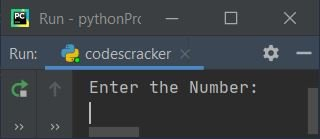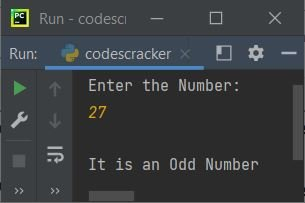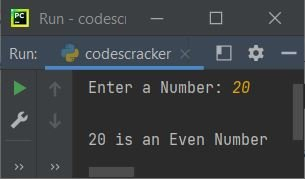# Python Program to Check Even or Odd Number

In this article, we've created some programs in Python, to check whether a number entered by user at run-time is an even or an odd number. Here are the list of programs:

• Simple Program to Check Even or Odd Number
• Check Even or Odd using single line if-else
• Using Function
• Using Class

## Check Even or Odd Number

The question is, write a Python program to check whether a given number is an even or an odd number. Here is its answer:

```print("Enter the Number: ")
num = int(input())
if num%2==0:
print("\nIt is an Even Number")
else:
print("\nIt is an Odd Number")```

Here is its sample run:Now supply the input say 27 and press `ENTER` key to check whether it is an even or an odd number:#### Modified Version of Previous Program

In this program, we've used end= to skip printing of an automatic newline using print(). And the method named str() is used to convert any type of value to a string type, because concatenation using + operator is only happened with similar type. Therefore we've converted the value of num to string:

```print("Enter a Number: ", end="")
num = int(input())

if num%2==0:
print("\n" +str(num)+ " is an Even Number")
else:
print("\n" +str(num)+ " is an Odd Number")```

Here is its sample run with user input 20:### Check Even or Odd Number using One-line if-else

This program uses single line if-else statement to check and print whether a number is an even or an odd:

```print("Enter a Number: ", end="")
num = int(input())

print("Even Number") if num%2==0 else print("Odd Number")```

Here is its sample run with user input, 2:## Check Even or Odd using Function

This program does the same job as of previous program, but using a user-defined function named checkEvenOdd(). This function takes the number entered by user as its argument and prints the message whether it is an even or an odd number:

```def checkEvenOdd(n):
print("Even Number") if n % 2 == 0 else print("Odd Number")

print("Enter a Number: ", end="")
num = int(input())

checkEvenOdd(num)```

#### Same Program with return Value

This program is similar to previous program. The only difference is, instead of printing the message from inside the function, the function returns the value 1, if number is even.

```def checkEvenOdd(n):
if n%2==0:
return 1

print("Enter a Number: ", end="")
num = int(input())

chk = checkEvenOdd(num)
if chk==1:
print("Even Number")
else:
print("Odd Number")```

## Check Even or Odd using Class

This is the last program, created using class, an object-oriented feature of Python to do the same job, that is checking for even or odd number.

```class CodesCracker:
def checkEvenOdd(self, n):
if n%2==0:
return 1

print("Enter a Number: ", end="")
num = int(input())

obj = CodesCracker()
chk = obj.checkEvenOdd(num)
if chk==1:
print("Even Number")
else:
print("Odd Number")```

An object named obj is created of CodesCracker() class. So this object can be used to access its member function named checkEvenOdd() using dot (.) operator. Rest of the things works like similar to a normal function.

#### Same Program in Other Languages

Python Online Test

« Previous Program Next Program »